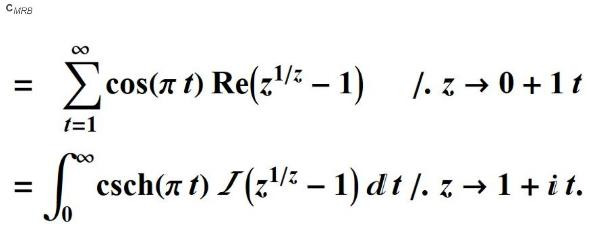For what value of x is f(x)=x^(1/x) a maximum?f'(x)=x^(1/x-2)(1-lnx)=0,         x=e and gives a maximum of e^(1/e)=1.444667861... Since         = 3, consider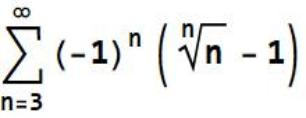. Given that C MRB =it follows that
 where A is the Glaisher-Kinkelin constant.
 user90369 (https://math.stackexchange.com/users/332823/user90369), High precision evaluation of the series $\sum_{n=3}^\infty (-1)^n (1-n^{1/n})$, URL (version: 2019-07-16): https://math.stackexchange.com/q/3294599
 CMRB from Steiner's Problem
 The MRB constant(CMRB).
 These first equations were first publishedin book form byFinch, Steven R. (2003). Mathematical Constants.Cambridge, England: Cambridge University Press. p. 450.ISBN 0-521-81805-2.
 The following formulas were first published in book form byCrandall, R. E. "The MRB Constant." §7.5 in Algorithmic Reflections: Selected Works. PSI Press, pp. 28-29, 2012b.They are an application of Fubini’s theorem for double series.
 The following identities are applications of the Abel-Plana formula,first published  by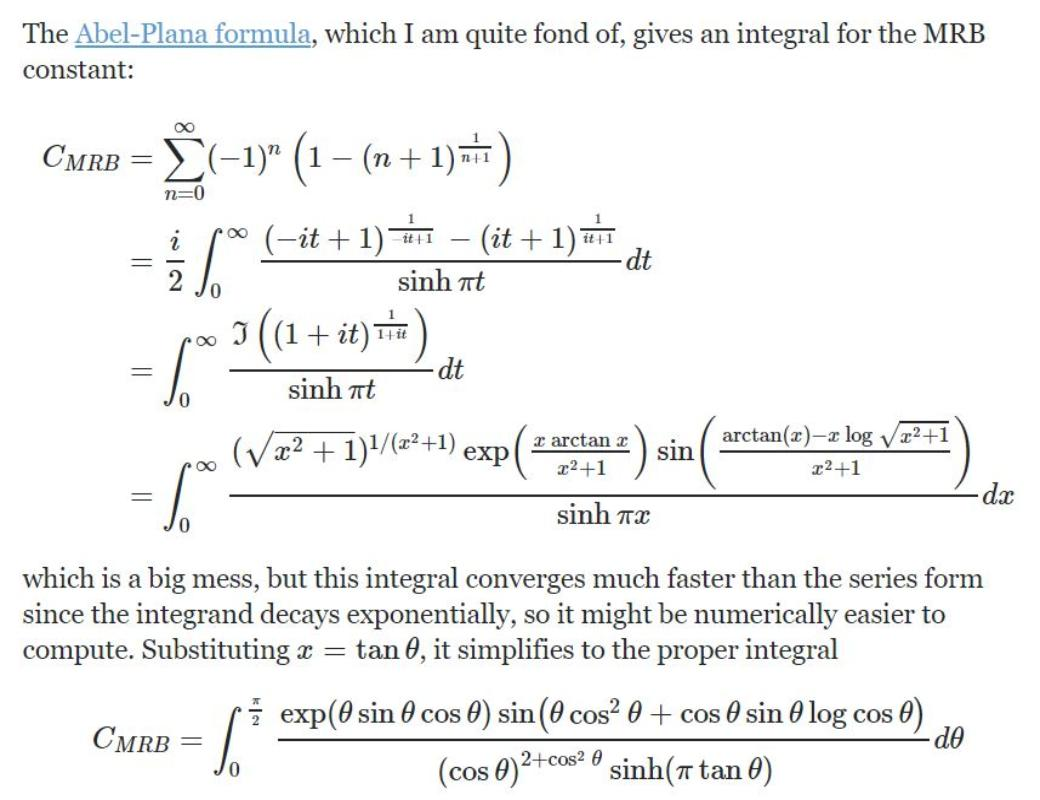The Online Encyclopedia of Integer Sequences oeis.org/wiki/MRB_constant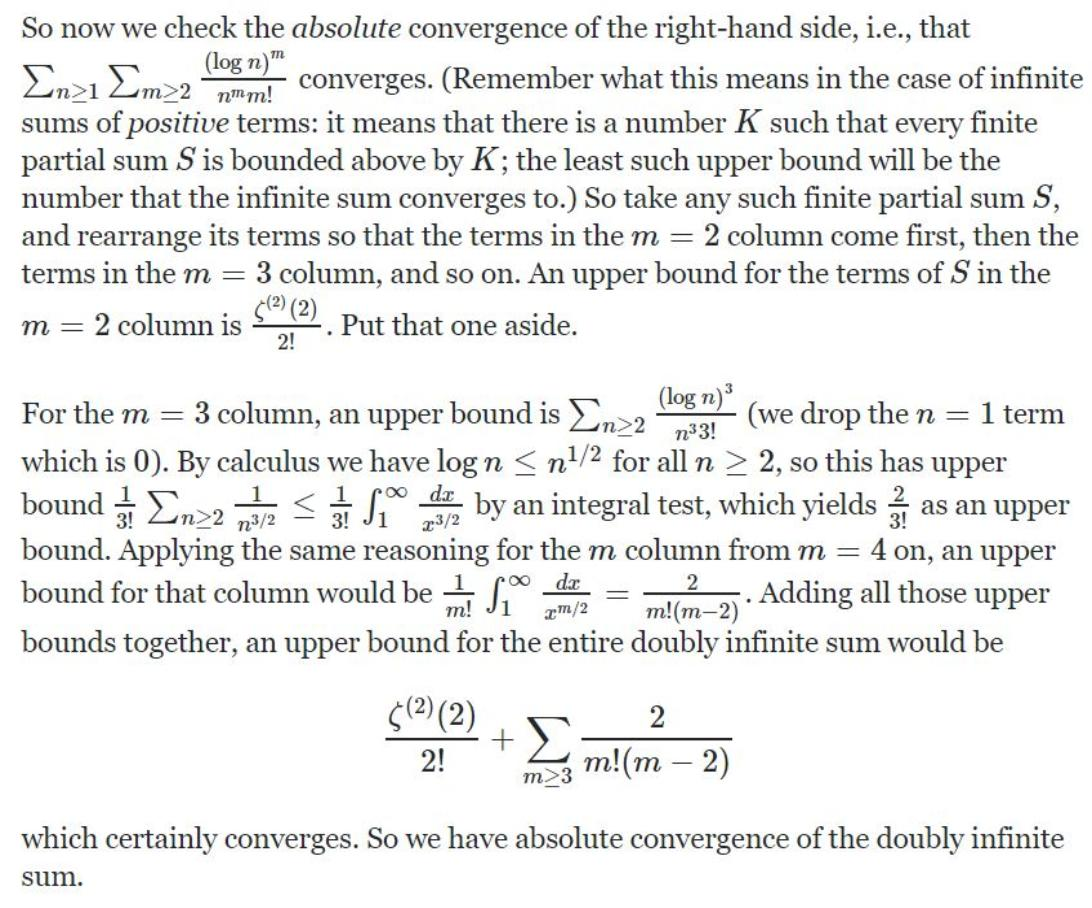Dark Malthorp (https://math.stackexchange.com/users/532432/dark-malthorp), What are some working models that are a fit the formula for the MRB constant?, URL (version: 2020-01-12): https://math.stackexchange.com/q/3505694
 5,555,555 digit computation of CMRB(MRB supercomputer output at 5:40:08 pm EDT  |  Monday, August 10, 2020)Start time was Fri 19 Jun 2020 22:20:08.Iterations required: 7369998Will give 3599 time estimates, each more accurate than the previous.Will stop at 7370752 iterations to ensure precision of around 5583332 decimal places....3166208 iter. done in 4.476*10^6 s. Ave. 0.70741iter./s. Should take 120.58 days or 1.042*10^7s. Finish Sun 18 Oct 2020 12:18:31.{42.9564,% done.}MRB supercomputerProcessors: Intel Core i9-9900K (5.0 GHz Turbo) (16-Thread) (8-Core) 3.6 GHz. CPU Boost: Stage 2: Overclock CPU - Up to 5.1GHz on All CPU Cores at 3200 Mhz RAM Extreme Cooling: H20: Stage 2: Corsair H115i PRO - 280mm Liquid CPU Cooler  with the following two exterior nodes: 4.7 GHz on all Intel 6 cores at 3000 MHz RAM and 4 cores of 3.6 GHz at 2400 MHz RAM!{{u -> -3.205281240093347156628042917392342206845495258334899739232149151500845258217206440409918401887943074},

{u -> -1.975955817063408761652299553542124207955844914033641475935228264550492865978094627264109660184110877},
{u -> -1.028853359952178482391753039155168552490590931630510790413956782402957713372878008212396966920395899},
{u ->  0.02332059641642379960870201829771316178976827597634872153712935185694600973532346147587251063387838184},
{u -> 1.028851065679287940491239061962065335882572934488973325479147532561027453451485653303655759243419286},
{u -> 1.975930036556044011032057974393627818460615746707424999683678154612112980778643821981630632223625195},
{u -> 3.377688794565491686010340135121834601964847740798723565007400061225550945863584207850080547920439455},
{u -> 4.218664066279720330451890569753298644162048163522884025276106357736553673659421134883269758349165422}}
or
u=infinity

The MRB constant is defined at http://mathworld.wolfram.com/MRBConstant.html.
After a lot of looking I found a connection between the MRB constant and applied math:

The MRB constant is ∑(−1)^k(k^(1/k)−1), and that k^(1/k)−1 is the interest rate
to multiply an investment k times in k periods -- as well as other growth models
involving the more general expression (1+k)^n --
since
((k^(1/k)−1)+1)^k|k∈Z+=k. and ((k^(1/n)−1)+1)^n|n∈Z+=k.

We can say, the result of summing, with alternating signs, the interest rate to multiply an investment k times in k
periods (or the equivalent growth model) could be the end "growth" rate resulting from growth, following decay,
Real life application
Geometry

The Geometry of the MRB Constant
Authors: Marvin Ray Burns
The MRB constant is the upper limit point of the sequence of
partial sums defined by S(x)=sum((- 1)^n*n^(1/n),n=1..x). The
goal of this paper is to show that the MRB constant is
geometrically quantifiable. To “measure” the MRB constant, we
will consider a set, sequence and alternating series of the nth
roots of n. Then we will compare the length of the edges of a
special set of hypercubes or ncubes which have a content of n.
(The two words hypercubes and n-cubes will be used
synonymously.) Finally, we will look at the value of the MRB
constant as a representation of that comparison, of the length of
the edges of a special set of hypercubes, in units of dimension 1/
(units of dimension 2 times units of dimension 3 times units of
dimension 4 times etc.). For an arbitrary example we will use
units of length/ (time*mass* …).
Comments: 8 Pages. This classic paper shows the utter
simplicity of the geometric description of the MRB constant (oeis.
org/A037077).
Submission history
[v1] 2016-09-06 19:08:36
Inquiries?
bmmmburns@sbcglobal.net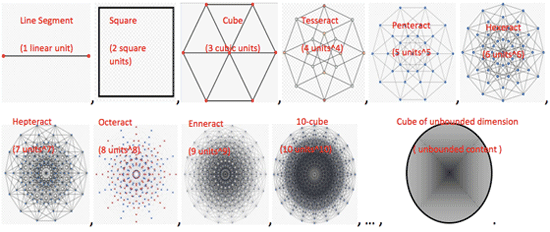{{u ->1.333754341654332447320456098329979657122884399228753780402849117971679458574998581211071632367720673},
{u -> 2.451894470180356539050514838856255986670981536525622143367559422740744344429034215340055686290476534}}
or
u=infinity
Or let x=1 and
 The MRB constant is related to the following oscillating divergent series
 Its partial sums
 are bounded within the closed interval
 and where the MRB constant is defined as
 since
 The MRB constant can be explicitly defined by the following infinite sums
 thus fulfilling the necessary and sufficient conditions for the convergence of an alternating series.
 where
 converges. (Remember what this means in the case of infinite sums of positive terms: it means that there is a number K such that every finite partial sum S is bounded above by K; the least such upper bound will be the number that the infinite sum converges to.) So take any such finite partial sum S, and rearrange its terms so that the terms in the m=2 column come first, then the terms in the m=3 column, and so on. An upper bound for the terms of S in the m=2 column is ζ(2)(2)2!. Put that one aside.
 So now we check the absolute convergence of the right-hand side, i.e., that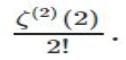which certainly converges. So we have absolute convergence of the doubly infinite sum.Thus we are in a position to apply the Fubini theorem, which justifies therearrangement expressed in the first of the following equations
 giving us what we wanted.
thenbounds together, an upper bound for the entire doubly infinite sum would be
 For the m=3 column, an upper bound is
 (we drop the n=1 term
 which is 0). By calculus we have
 for all n≥2, so this has upper
 bound
 by an integral test, which yields
 as an upper
 bound. Applying the same reasoning for the m column from m=4 on, an upper
 bound for that column would be
Let x=25.65665403510586285599072933607445153794770546058072048626118194900973217186212880099440071247391598 and
I discovered the following via the Mathematica notebook to the right. ->->Integrated analog of the CMRB seriesThe integrated analog of the series is a complex-valued integral of oscillatory character Not convergent in the continuum limit at  ,         the limit of the sequence of integrals with an integral difference in the upper limits 2n  exists.Ultraviolet limit MI of the sequence of oscillatory integralsThe ultraviolet limit of the sequence of oscillatory integrals is defined as and has been evaluated by Richard J. Mathar.The decimal expansion of the real part of MI isThe decimal expansion of the imaginary part of MI is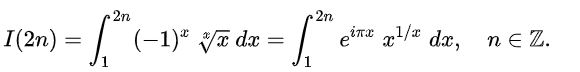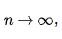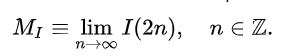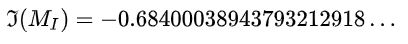The MRB constant notebook to the right. ->->

m=the MRB constant. We looked at how n^m-m is similar to E^Pi-Pi (a near integer). One might think this is off the subject of breaking computational records of
the MRB constant, but it also could help show whether there exists a closed-form for computing and checking the digits of m from n^m-m=a near integer and n
is an integer.

So, I decided to make an extremely deep search of the n^m-m=a near integer, and n is an integer field. Here are the pearls I gleaned:

In:= m =
NSum[(-1)^n (n^(1/n) - 1), {n, 1, Infinity}, WorkingPrecision -> 100,
Method -> "AlternatingSigns"];

In:= 225897077238546^m - m

Out= 496.99999999999999975304752932252481772179797865

In:= 1668628852566227424415^m - m

Out= 9700.9999999999999999994613109586919797992822178

In:= 605975224495422946908^m - m

Out= 8019.9999999999999999989515156294756517433387956

In:= 3096774194444417292742^m - m

Out= 10896.0000000000000000000000096284579090392932063

In:= 69554400815329506140847^m - m

Out= 19549.9999999999999999999999991932013520540825206

In:= 470143509230719799597513239^m - m

Out= 102479.000000000000000000000000002312496475978584

In:= 902912955019451288364714851^m - m

Out= 115844.999999999999999999999999998248770510754951

In:= 2275854518412286318764672497^m - m

Out= 137817.000000000000000000000000000064276966095482

In:= 2610692005347922107262552615512^m - m

Out= 517703.00000000000000000000000000000013473353420

In:= 9917209087670224712258555601844^m - m

Out= 665228.00000000000000000000000000000011062183643

In:= 19891475641447607923182836942486^m - m

Out= 758152.00000000000000000000000000000001559954712

In:= 34600848595471336691446124576274^m - m

Out= 841243.00000000000000000000000000000000146089062

In:= 543136599664447978486581955093879^m - m

Out= 1411134.0000000000000000000000000000000035813431

In:= 748013345032523806560071259883046^m - m

Out= 1498583.0000000000000000000000000000000031130944

In:= 509030286753987571453322644036990^m - m

Out= 1394045.9999999999999999999999999999999946679646

In:= 952521560422188137227682543146686124^m - m

Out=5740880.999999999999999999999999999999999890905129816474332198321490136628009367504752851478633240

In:= 50355477632979244604729935214202210251^m - m

Out=12097427.00000000000000000000000000000000000000293025439870097812782596113788024271834721860892874

In:= 204559420776329588951078132857792732385^m - m

Out=15741888.99999999999999999999999999999999999999988648448116819373537316944519114421631607853700001

In:= 4074896822379126533656833098328699139141^m - m

Out= 27614828.00000000000000000000000000000000000000001080626974885195966380280626150522220789167201350

In:= 100148763332806310775465033613250050958363^m - m

Out= 50392582.999999999999999999999999999999999999999998598093272973955371081598246

In=  116388848574396158612596991763257135797979^m - m

Out=51835516.000000000000000000000000000000000000000000564045501599584517036465406

In:= 111821958790102917465216066365339190906247589^m - m

Out= 188339125.99999999999999999999999999999999999999999999703503169989535000879619

In := 8836529576862307317465438848849297054082798140^m - m

Out = 42800817.00000000000000000000000000000000000000000000000321239755400298680819416095288742420653229

In := 532482704820936890386684877802792716774739424328^m - m

Out =924371800.999999999999999999999999999999999999999999999998143109316148796009581676875618489611792

In:= 783358731736994512061663556662710815688853043638^m - m

Out= 993899177.0000000000000000000000000000000000000000000000022361744841282020

In:= 8175027604657819107163145989938052310049955219905^m - m

Out= 1544126008.9999999999999999999999999999999999999999999999999786482891477\
944981

19779617801396329619089113017251584634275124610667^m - m
gives
1822929481.00000000000000000000000000000000000000000000000000187580971544991111083798248746369560.

130755944577487162248300532232643556078843337086375^m - m

gives

2599324665.999999999999999999999999999999999999999999999999999689854836245815499119071864529772632.
i.e.2, 599, 324, 665. 999, 999, 999, 999, 999, 999, 999, 999, 999, 999, 999, 999, 999, 999, 999, 999, 999, 689

(51 consecutive 9 s)

322841040854905412176386060015189492405068903997802^m - m

gives

3080353548.000000000000000000000000000000000000000000000000000019866002281287395703598786588650156

i.e. 3, 080, 353, 548. 000, 000, 000, 000, 000, 000, 000, 000, 000, 000, 000, 000, 000, 000, 000, 000, 000,019

(52 consecutive 0 s)

310711937250443758724050271875240528207815041296728160^m - m

gives

11195802709.99999999999999999999999999999999999999999999999999999960263763...
i.e. 11,195,802,709. 999, 999, 999, 999, 999, 999, 999, 999, 999, 999, 999, 999, 999, 999, 999, 999, 999, 999, 602, 637,63

(55 consecutive 9s)

1465528573348167959709563453947173222018952610559967812891154^ m - m
gives
200799291330.9999999999999999999999999999999999999999999999999999999999999900450730197594520134278
i. e 200, 799, 291, 330.999, 999, 999, 999, 999, 999, 999, 999, 999, 999, 999, 999, 999, 999, 999, 999, 999, 999, 999, 999, 99

(62 consecutive 9 s).
I did a little experimenting and found that the existence of such precise near integers from n^m-m is not specific to the MRB constant due to any internal
quality, only due to the small value of m. You can find similarly precise approximations for n^x-x, where x is 1/E. 1/E(.37) has a value in the same neighborhood
of the MRB constant (.19).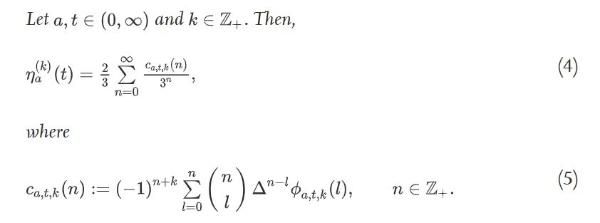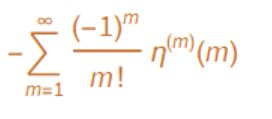CMRB=
1
 OPEN QUESTION. Which algorithm is faster?
OR
WHERE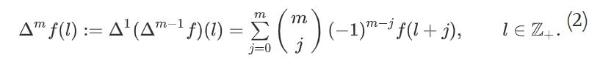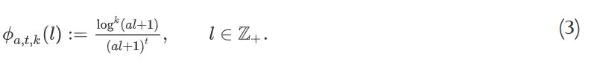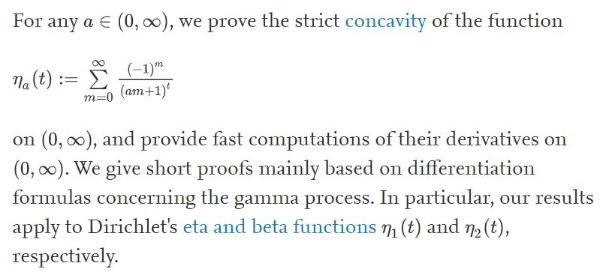1
 user43208 (https://math.stackexchange.com/users/43208/user43208), Is there a more rigorous way to show these two sums are exactly equal?, URL (version: 2016-02-28): https://math.stackexchange.com/q/1675189ORWHERE
CMRB=